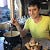As the covid-19 pandemic fades in the US, many companies are preparing their employees to return to the office. …

# Leetcode 1876: Substrings of Size Three with Distinct Characters

In this problem (https://leetcode.com/problems/substrings-of-size-three-with-distinct-characters/), we want to count the number of substring of length 3(i.e. 3 characters in a row in the string), such that these substring don’t contain duplicate characters.

Given n the length of the input string, we can solve the problem by testing all the substrings of…

# Leetcode 1793: Maximum Score of a Good Subarray

In this problem, we are trying to optimize the “score” of “good subarrays defined this way:

You are given an array of integers `nums` (0-indexed) and an integer `k`.

The score of a subarray `(i, j)` is defined as `min(nums[i], nums[i+1], ..., nums[j]) * (j - i + 1)`. …

# Leetcode 1791: Find center of Star Graph

In this Leetcode problem, we are given a list of edges representing a star graph. A star graph is a graph where all the edges contain the “center” node. This is the problem statement:

There is an undirected star graph consisting of `n` nodes labeled from `1` to `n`. A…

# Solution to Leetcode 1749. Maximum Absolute Sum of Any Subarray

This problem is very classic. I find it helpful to see all the values in the array, as differences between values in a series, and to draw it on paper. Don’t try to find a complicated solution to this problem. You only need to remember a couple of things like…

# Leetcode 1704: Determine if String Halves Are Alike. All main languages

In this Leetcode problem, we need to count the vowels in each half of the string in input. If the number of vowels is the same in both halves, we return true, we return false otherwise.

Here is the implementation for the all the main backend languages, in alphabetical order:

`…`

# Leetcode 1679: Max Number of K-Sum Pairs

In this Leetcode problem, we are given an array `nums` of integers. In one operation, we can take any pair of integers adding up to `k` and take it out of the array. We want to know the maximum number of operations that we can do following that rule.

For…

# Leetcode 1670: Design Front Middle Back Queue

In this Leetcode problem, we are implementing a Queue from from with we can push and pop from the front, back, or middle.

## Solution

Obviously, the pop and push from the middle are the core of the problem. Otherwise we could use a LinkedList.

To solve this problem, we break up…

# Leetcode 1669: Merge In Between Linked Lists

In this Leetcode problem, we want to merge a list `list2` into another list `list1` and remove some elements of `list1` where `list2` is going.

We want to simply build the list simply by going to the node in `list1` where `list2` should be inserted. …
In this Leetcode problem, we are given a String representing a list of keys pressed on a keyboard. We are given the time for which each key was released in the array `int[] releaseTimes`. We assume that at the exact same time that a key is released, the next key…